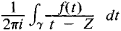Cauchy Integral

(redirected from Cauchy's integral theorem)
Also found in: Wikipedia.

Cauchy Integral

an integral of the formwhere γ is a simple closed rectifiable curve in a complex plane and f(t) is a function of the complex variable t analytic on γ and in the interior of γ. If the point z lies within γ, then the Cauchy integral is equal to f(z). Thus, the Cauchy integral expresses the values of the analytic function f inside γ in terms of the values of f on γ. The integral was first examined by A. Cauchy in 1831.

Cauchy-type integrals are generalizations of the Cauchy integral. They have the same form, but the curve γ is not assumed to be closed and the function f(t) is not assumed to be analytic. Such integrals also define analytic functions. Their values on γ generally differ from the function f(t).

The systematic study of these integrals was begun by I. V. Sokhotskii and was subsequently continued chiefly by Russian and Soviet mathematicians (Iu. G. Kolosov, V. V. Golubev, I. I. Privalov, and N. I. Muskhelishvili). These studies led to further generalizations as well as to applications to problems of mechanics.

REFERENCES

Markushevich, A. I. Teoriia analiticheskikh funktsii, 2nd ed., vols. 1–2. Moscow, 1967–68.
Privalov, I.I. Granichnye svoistva analiticheskikh funktsii, 2nd ed. Moscow-Leningrad, 1950.
Site: Follow: Share:
Open / Close